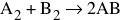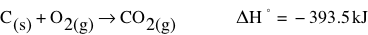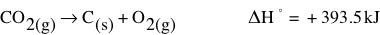# Bond Energy

In our study of bonding, you already learned that in order for a bond to form, there must be a lowering of energy. This can only happen if energy is released. You can think of the individual atoms as having energy and have to be slowed down so that chemical bonds can form. Thus, making chemical bonds releases energy. The reverse is true for the formation of bonds. In order to break a chemical bond, energy must be put into the system to overcome the attractive interaction between the shared electrons and the nuclei of the bonded atoms.

Breaking bonds requires energy, and making bonds releases energy.Relating this idea to that of internal energy, we know that internal energy is a function of the heat and work that goes in and out of the system. Since most chemical transformations do not involve work, the internal energy is mostly a function of the heat that enters or leaves the system. So, when a chemical bond is formed, heat leaves the system, lowering the internal energy of the system. Work must be done to separate atoms in a bond.

The amount of energy needed to break the bond is called the bond energy.

Lots of heat and/or light energy is released when very strong bonds form, because much of the chemical energy is converted to heat and/or light energy. The diagram to the right shows how a chemical reaction converts chemical energy (the potential energy of the atoms) into heat and light energy. The reverse is true for breaking chemical bonds. It takes more energy to break a strong bond than a weak bond. The breaking of a bond requires the absorption of heat and/or light energy which is converted into chemical energy when the bond is broken.

This concept is frequently misunderstood by students. Read the article Ending Misconceptions About the Energy of Chemical Bonds on the College Board AP Central website for more insight on this idea.

## Endothermic/Exothermic

Chemical reactions are a combination of bond breaking and bond formation. The overall reaction can be classified by considering the energy change by the total energy required (to break bonds) and the total energy released (by the formation of bonds). If the total energy released is greater than the total energy required, then the reaction is exothermic. If the total energy required is greater than the total energy released, the reaction is endothermic.

In an exothermic chemical reaction, the electrons and nuclei within the reactants undergo rearrangement into products that possess lower total energy. The energy lost by the reacting molecules (system) is gained by the surroundings. The energy is transferred to the surroundings by either heat or work. Likewise, for endothermic reactions, the system gains energy from the surroundings by heat transfer or work done on the system. In this case, the system has a positive value of q.

Processes that are not chemical reactions, like phase changes, can be classified as endothermic or exothermic as well.One way to tell if a process or reaction is endothermic or exothermic is to measure the change in temperature. If it is endothermic, the temperature will decrease. If it is exothermic, the temperature will increase. This might seem counterintuitive to you. If an endothermic reaction absorbs heat, wouldn’t the temperature increase? No! When an endothermic reaction absorbs heat, that energy is used for the reaction itself, leaving the surroundings with a lower temperature. Remember that in an isolated system, energy is conserved. In an endothermic process, the potential energy increases. This means that the kinetic energy must decrease. And, what does temperature measure? It measures the average KE! Noticing the change in temperature of a reaction is an easy way to determine if it is exothermic or endothermic.

## EnthalpyIn order to calculate the changes in energy associated with chemical reactions, we need to introduce a new term, enthalpy or H. It can also be referred to as heat content. Most reactions that we observe and perform in the lab take place in containers that are open to the atmosphere, where the pressure remains essentially constant over a short period of time. The equation for change in internal energy, ∆U = q + w , can be modified to indicate this constant pressure. The modified equation is H = E + PV or at constant pressure, ∆H = qp (qp is heat at constant pressure). Look in your book to see the complete derivation of enthalpy. Enthalpy change is the name given to the amount of heat evolved or absorbed in a reaction carried out at constant pressure. It is just a more specific term than q. It is given the symbol ΔH, read as “delta H” and is measured in kJ/mol.

When a system absorbs energy from the surroundings, an endothermic reaction, Hproducts is larger than Hreactants, so ΔH is positive.

When a system loses energy to the surroundings, an exothermic reaction, Hproductsis smaller than Hreactants, so ΔH is negative.

The following interactive from shows that enthalpy change in a reaction is associated with the differences between the bond strengths (bond enthalpy) of the reactant and the product molecules. This simulation allows the bond energy of the three simple molecules to be adjusted. The overall reaction isAnd the enthalpy change, ∆H, for the reaction is indicated.

## Standard Heat of Reaction

The ΔH for any reaction depends upon the number of moles of reactants you start with. It should make sense that burning 2 mol of C would release twice as much energy as burning 1 mol of C. Because other factors can affect ΔH as well, chemists have agreed to a set of standards to use to measure ΔH. The standard conditions include a pressure of 1 atm, a concentration of 1 M (for aqueous substances), and a temperature of 25oC (298 K). Note that this temperature is different than the standard temperature for gas law problems (Recall that STP means a standard temperature of 0 oC. So why do we have a different standard temperature here? It is because 25oC represents average room temperature where these ΔH values are measured.) To indicate that a heat of reaction is the standard heat of reaction, the symbol has a degree sign added, ΔH°.

A chemical equation that is written to show the heat of reaction is called a thermochemical equation. These can be written for any heats of reaction, standard or not. The thermochemical equation below represents the combustion of carbon in oxygen.This thermochemical equation can be reversed. In the reverse reaction, the value of ΔH° is the opposite sign.If we double the number of moles of each chemical, we double the value of ΔH°as well.Based on the following, write the thermochemical reaction for the decomposition of 4 moles of ammonia.

N2 + 3H2 → 2NH3
ΔH = -40.9 kJ

Flip the reaction, changing the negative to positive.
2NH3 → N2 + 3H2
ΔH = +40.9 kJ

Then, double the entire reaction.
4NH3 →2N2 + 6H2
ΔH = +81.8 kJ

It is important to relate what you learn to your previous knowledge. Read the handout in the sidebar that explains the relationship between bond energy, bond strength and length (that you learned about in the bonding module), and enthalpy.

## Standard Heat of Reaction

The ΔH for any reaction depends upon the number of moles of reactants you start with. It should make sense that burning 2 mol of C would release twice as much energy as burning 1 mol of C. Because other factors can affect ΔH as well, chemists have agreed to a set of standards to use to measure ΔH. The standard conditions include a pressure of 1 atm, a concentration of 1 M (for aqueous substances), and a temperature of 25oC (298 K). Note that this temperature is different than the standard temperature for gas law problems   (Recall that STP means a standard temperature of 0   o C.   So why do we have a different standard temperature here? It is because 25oC represents average room temperature where these ΔH values are measured.)   To indicate that a heat of reaction is the standard heat of reaction, the symbol has a degree sign added, ΔH°.

source